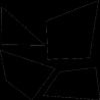#### You may also like### Framed

Seven small rectangular pictures have one inch wide frames. The frames are removed and the pictures are fitted together like a jigsaw to make a rectangle of length 12 inches. Find the dimensions of the pictures.### Tilted Squares

It's easy to work out the areas of most squares that we meet, but what if they were tilted?### From One Shape to Another

Read about David Hilbert who proved that any polygon could be cut up into a certain number of pieces that could be put back together to form any other polygon of equal area.

# Four or Five

##### Age 11 to 14 ShortChallenge Level

Using the options given
450 $\div$ 9 = 50
50 = length $\times$ width, where length : width = 5 : 4
50 = 5 $\times$ 10
No closer factor pairs $\therefore$ no factor pairs in the ratio 5 : 4

1260 $\div$ 9 = 140
140 = 2 $\times$ 70
= 4 $\times$ 35
= 10 $\times$ 15
No closer factor pairs $\therefore$ no factor pairs in the ratio 5 : 4

1620 $\div$ 9 = 180
180 = 10 $\times$ 18
= 12 $\times$ 15 correct ratio
So 1620 is possible

Using $x$
The ratio length:breadth of the smaller rectangles is $5:4$. Let the length and breadth of these rectangles be $5x \; \text{cm}$ and $4x\; \text{cm}$ respectively. The area of the large rectangle, in cm$^2$, is $9 \times 20x^2 = 180x^2$ and the only one of the alternatives which is a product of $180$ and a perfect square is $1620$, which corresponds to $x = 3$.

This problem is taken from the UKMT Mathematical Challenges.
You can find more short problems, arranged by curriculum topic, in our short problems collection.